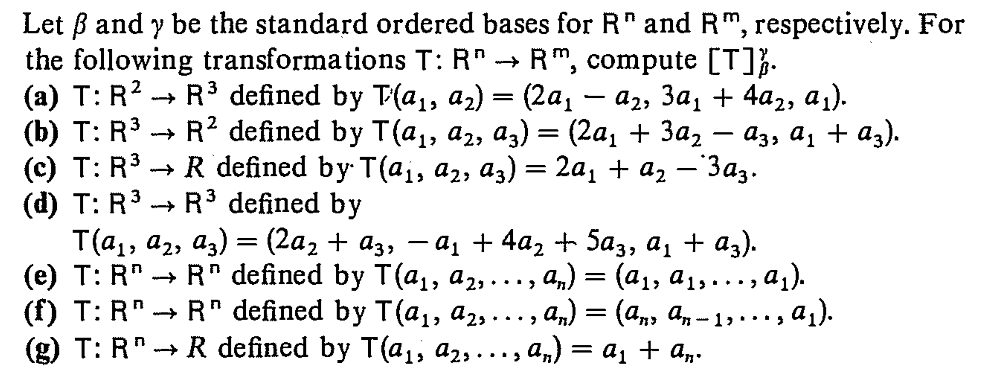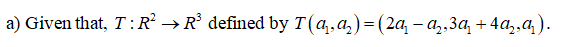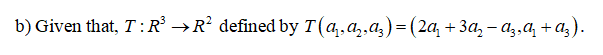# Let ß and y be the standard ordered bases for R" and R", respectively. Forthe following transformations T: R* → R", compute [T]}.(а) Т: R? — R3 defined by T(а,, а2) — (2а, — а,, За, + 4а,, а).(b) T: R3(c) T: R3(d) T: R3T(а, а,, аз) — (2а, + aз, — а, + 4a, t 5аз, а, + a).(е) Т: R" > R" defined by T (ај, а2, .., а,) 3D (ај, ај, ...,a).(() T:R" — R" defined by T(aj, аz, ..., an) 3 (ал, а, - 13 .., аj).(g) T: R" -R? defined by T(а,, а, аз) — (2а, + За, — аза а, + ag).> R defined bу T(а;, а2, а,) — 2а, + a, — Заз-R3 defined byR defined by T(a1, a2,..., a,) = a1 + a„.

Question
10 viewshelp_outlineImage TranscriptioncloseLet ß and y be the standard ordered bases for R" and R", respectively. For the following transformations T: R* → R", compute [T]}. (а) Т: R? — R3 defined by T(а,, а2) — (2а, — а,, За, + 4а,, а). (b) T: R3 (c) T: R3 (d) T: R3 T(а, а,, аз) — (2а, + aз, — а, + 4a, t 5аз, а, + a). (е) Т: R" > R" defined by T (ај, а2, .., а,) 3D (ај, ај, ...,a). (() T:R" — R" defined by T(aj, аz, ..., an) 3 (ал, а, - 13 .., аj). (g) T: R" - R? defined by T(а,, а, аз) — (2а, + За, — аза а, + ag). > R defined bу T(а;, а2, а,) — 2а, + a, — Заз- R3 defined by R defined by T(a1, a2,..., a,) = a1 + a„. fullscreen
check_circle

Step 1

Hey since there are multiple subparts the first three subparts is answered if any subpart has to be answered kindly repost that particular question.

Step 2Step 3...

### Want to see the full answer?

See Solution

#### Want to see this answer and more?

Solutions are written by subject experts who are available 24/7. Questions are typically answered within 1 hour.*

See Solution
*Response times may vary by subject and question.
Tagged in

### Other